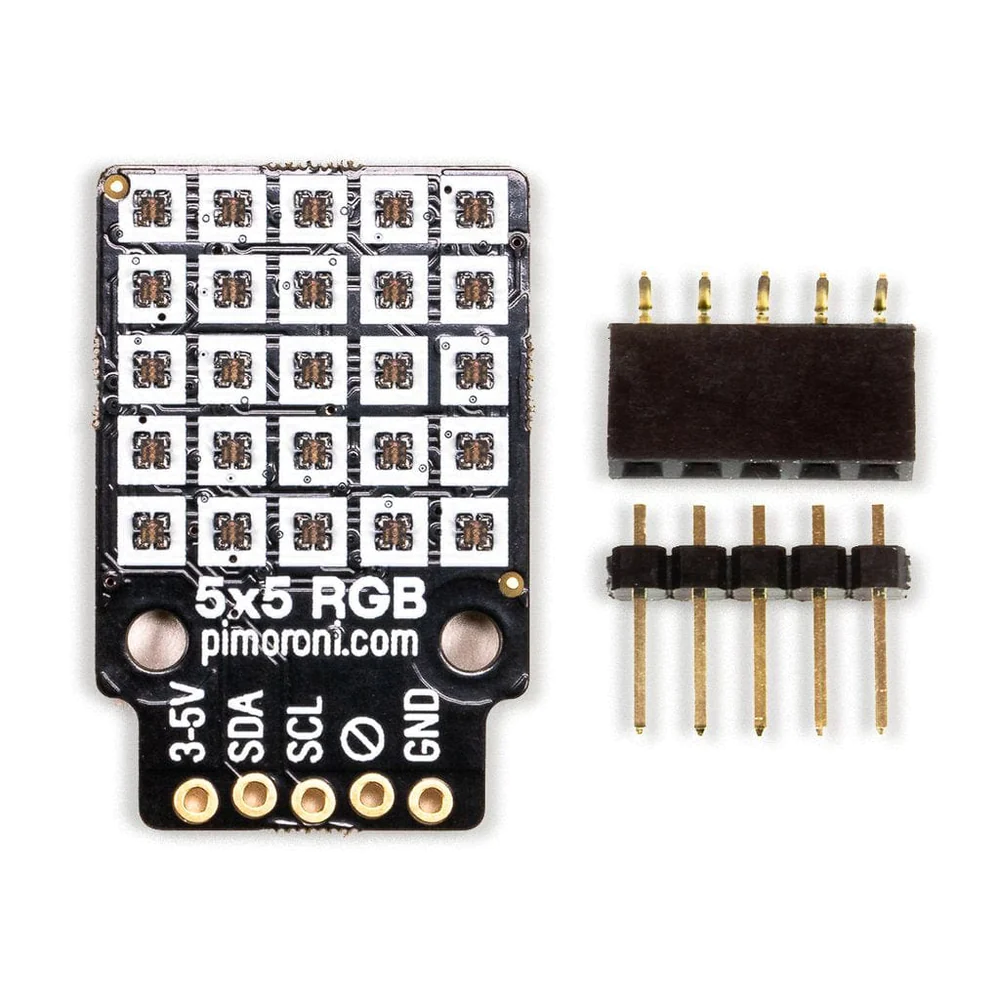Home CodeMicropython Raspberry Pi Pico and 5×5 RGB Led matrix micropython example

# Raspberry Pi Pico and 5×5 RGB Led matrix micropython example

In this article we connect a 5×5 RGB Led matrix from Pimoroni to the Raspberry Pi Pico

### Breakout Information## Features

• 5×5 (25 total) RGB LEDs
• 15x15mm active area
• 3.5mm LED pitch
• I2C interface (address 0x74/0x77 (cut trace))
• 3.3V or 5V compatible
• Reverse polarity protection

### Parts Required

The sensor you can pick up in the \$6 price range – you can connect to the sensor using a standard header the classic dupont style jumper wire.

I used a Qwiic cable – since a few sensors seem to use these but this is optional

 Name Link Pico Raspberry Pi Pico Development Board 5×5 RGB Led matrix https://shop.pimoroni.com/products/5×5-rgb-matrix-breakout Connecting cables Aliexpress product link Lysee 3D Printer Parts & Accessories – AHT20 Temperature and Humidity Sensor Module DHT11 Upgrade I2C XD Humidity Sensor Probe – (Color: Green) Ebay link### Schematic/Connection

I used the Pimoroni 5×5 RGB Led matrix

### Code Examples

I used Thonny for development and I am using micropython

You will need to download and install the latest micropython from pimoro

https://github.com/pimoroni/pimoroni-pico/blob/main/setting-up-micropython.md

```import time
from pimoroni_i2c import PimoroniI2C
from breakout_rgbmatrix5x5 import BreakoutRGBMatrix5x5

PINS_BREAKOUT_GARDEN = {"sda": 4, "scl": 5}
PINS_PICO_EXPLORER = {"sda": 20, "scl": 21}

i2c = PimoroniI2C(**PINS_PICO_EXPLORER)
matrix = BreakoutRGBMatrix5x5(i2c)

x = 0
y = 0
col = 0

while True:
matrix.set_pixel(x, 1, 255, 0, 0)
matrix.update()
x += 1
if x >= matrix.WIDTH:
x = 0
time.sleep(0.01)```

```import time
from pimoroni_i2c import PimoroniI2C
from breakout_rgbmatrix5x5 import BreakoutRGBMatrix5x5

PINS_BREAKOUT_GARDEN = {"sda": 4, "scl": 5}
PINS_PICO_EXPLORER = {"sda": 20, "scl": 21}

i2c = PimoroniI2C(**PINS_PICO_EXPLORER)
matrix = BreakoutRGBMatrix5x5(i2c)

x = 0
y = 0
col = 0

while True:
matrix.set_pixel(1, y, 255, 0, 0)
matrix.update()
y += 1
if y >= matrix.HEIGHT:
y = 0
time.sleep(0.01)```

```import time
from pimoroni_i2c import PimoroniI2C
from breakout_rgbmatrix5x5 import BreakoutRGBMatrix5x5

PINS_BREAKOUT_GARDEN = {"sda": 4, "scl": 5}
PINS_PICO_EXPLORER = {"sda": 20, "scl": 21}

i2c = PimoroniI2C(**PINS_PICO_EXPLORER)
matrix = BreakoutRGBMatrix5x5(i2c)

x = 0
y = 0
col = 0

while True:
matrix.set_pixel(1, 1, 255, 0, 0)
matrix.set_pixel(2, 2, 0, 255, 0)
matrix.update()```

```import time
from pimoroni_i2c import PimoroniI2C
from breakout_rgbmatrix5x5 import BreakoutRGBMatrix5x5

PINS_BREAKOUT_GARDEN = {"sda": 4, "scl": 5}
PINS_PICO_EXPLORER = {"sda": 20, "scl": 21}

i2c = PimoroniI2C(**PINS_PICO_EXPLORER )
matrix = BreakoutRGBMatrix5x5(i2c)

colors = []
colors.append((255, 0, 0))
colors.append((0, 255, 0))
colors.append((0, 0, 255))
colors.append((128, 128, 128))

x = 0
y = 0
col = 0

while True:
matrix.set_pixel(x, y, colors[col], colors[col], colors[col])
matrix.update()

x += 1
if x >= matrix.WIDTH:
x = 0
y += 1
if y >= matrix.HEIGHT:
y = 0
col += 1
if col >= len(colors):
col = 0
time.sleep(0.5)

time.sleep(0.01)```